# Fractions + triangle - math problems

#### Number of problems found: 28

• Infinite sum of areasAbove the height of the equilateral triangle ABC is constructed an equilateral triangle A1, B1, C1, of the height of the equilateral triangle built A2, B2, C2, and so on. The procedure is repeated continuously. What is the total sum of the areas of all tr
• Triangle in circle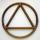Vertices of the triangle ABC lies on a circle with radius 3 so that it is divided into three parts in the ratio 4:4:4. Calculate the circumference of the triangle ABC.
• The angles ratioThe angles in the ABC triangle are in the ratio 1: 2: 3. find the sizes of the angles and determine what kind of a triangle it is.
• The angles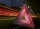The angles in the triangle are in the ratio 12: 15: 9. Find the angles.
• Similar trianglesIn the triangle DEF is DE = 21cm, EF = 14.7cm, DF = 28cm. The triangle D´E´F´ is similar to the triangle DEF. Calculate the lengths of the sides of the triangle D´E´F´ if the similarity coefficient is one-seventh.
• Equilateral triangle ABCIn the equilateral triangle ABC, K is the center of the AB side, the L point lies on one-third of the BC side near the point C, and the point M lies in the one-third of the side of the AC side closer to the point A. Find what part of the ABC triangle cont
• Circumferential angleVertices of the triangle ΔABC lies on circle and divided it into arcs in the ratio 2:2:9. Determine the size of the angles of the triangle ΔABC.
• Interior anglesCalculate the interior angles of a triangle that are in the ratio 2: 3: 4.
• Right triangle from axesA line segment has its ends on the coordinate axes and forms with them a triangle of area equal to 36 square units. The segment passes through the point ( 5,2). What is the slope of the line segment?
• Medians 2:1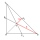Median to side b (tb) in triangle ABC is 12 cm long. a. What is the distance of the center of gravity T from the vertex B? b, Find the distance between T and the side b.
• MO Z9–I–2 - 2017In the VODY trapezoid, VO is a longer base and the diagonal intersection K divides the VD line in a 3:2 ratio. The area of the KOV triangle is 13.5 cm2. Find the area of the entire trapezoid.
• BevelI have bevel in the ratio 1:6. What is the angle and how do I calculate it?
• Triangular prismThe base of the perpendicular triangular prism is a rectangular triangle with a hypotenuse of 10 cm and one leg of 8 cm. The prism height is 75% of the perimeter of the base. Calculate the volume and surface of the prism.
• Above EarthTo what height must a boy be raised above the earth in order to see one-fifth of its surface.
• Painters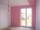Six of the painters paint 90 m of fence in five hours. For how long would the 4 painters paint a 45 meter fence? How many meters fence painted painters 5 for two hours?
• RiverCalculate how many permille river Dunaj average falls, if on section long 957 km flowing water from 1454 m AMSL to 101 m AMSL.
• Lookout towerCalculate the height of a lookout tower forming a shadow of 36 m if at the same time a column 2.5 m high has a shadow of 1.5 m.
• The pole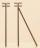The telegraph pole is supported by a 4 m bullet at 3/4 of its height, the end of which is at a distance 2.5 m from the pole post. Calculate the height of the telegraph pole.
• Castle towerThe castle tower has a cone-shaped roof with a diameter of 10 meters and a height of 8 meters. Calculate how much m² of coverage is needed to cover it if we must add one-third for the overlap.
• Trapezoid MOThe rectangular trapezoid ABCD with the right angle at point B, |AC| = 12, |CD| = 8, diagonals are perpendicular to each other. Calculate the perimeter and area of ​​the trapezoid.

Do you have an interesting mathematical word problem that you can't solve it? Submit a math problem, and we can try to solve it.

We will send a solution to your e-mail address. Solved examples are also published here. Please enter the e-mail correctly and check whether you don't have a full mailbox.

Please do not submit problems from current active competitions such as Mathematical Olympiad, correspondence seminars etc...